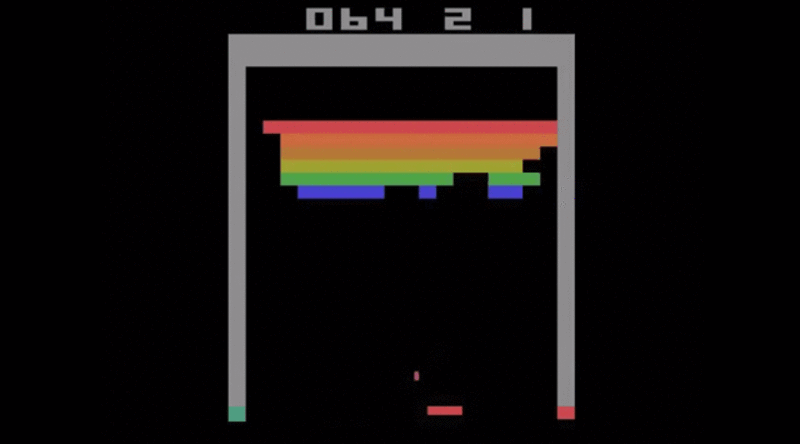June 13, 2016

# Teach an AI to Play Breakout: Curriculum & Lessons | Bonsai Blog###### This is part two in a series of blog posts detailing how we trained an AI to play Breakout using Bonsai’s platform. We recommend going back and starting here.

Let’s do a quick recap, we designed schemas to represent our input (a grayscale image of the board) and the output (playermove). We also used concepts to encode learning into our mental model.

In this post, we’re going to talk about how we use the concepts that we’ve codified in Inkling to actually teach the AI. We do this using a curriculum. A curriculum defines the data or simulation used to teach a concept; every designated concept will have a curriculum from which to learn. And for every curriculum, we will have one or more lessons. Continuing our analogy about teaching math to children, curriculums are analogous to an algebra textbook, and the lessons are individual chapters within the textbook, broken into smaller components in order to teach ideas bit-by-bit rather than all at once.

## Lets dive into some Inkling code to understand how it all works:

### get_high_score_curriculum

We will write a curriculum to train the model on the get_high_score.

curriculum get_high_score_curriculum     train get_high_score     with simulator breakout_simulator(BreakoutConfig):(GameState)     objective score

For every specified concept, we need to write a curriculum. The curriculum keyword declares a set of lessons, each declared by the lesson keyword, used to teach a concept. The train keyword indicates which concept this curriculum trains, the with keyword specifies which simulation (or data) should be used with this curriculum, and the objective keyword specifies the goal used to evaluate the learning system’s performance.

In the above snippet, the get_high_score_curriculum trains the concept get_high_score using the Breakout simulator which can be written in any language (in our case, we used an open-source simulator written in python). The objective of the above curriculum is to increase the score as much as possible.

Every curriculum will have one or more lessons. Lessons give the programmer control over the training of the model. Here is the example of a lesson for the get_high_score_curriculum

lesson score_lesson    configure         constrain bricks_percent=0.5,         constrain level=1,         constrain paddle_width=4    until         maximize scoreend

In this example, we constrain the simulator to certain parameters and specify the maximize keyword that directs the AI to maximize the objective of the curriculum. The author of the simulator defines both the objective and which parameters can be constrained using Bonsai’s Python SDK.

When the programmer is ready to train the AI, they connect the Breakout simulator to the server and issue a command to begin training. Once training starts, the server configures and plays the simulator until the AI agent maximizes the score as best it can or training is terminated.  Simulators can be reused to teach multiple concepts. Let’s see an example of reusing the breakout simulator to train the ball_location concept.

### ball_location_curriculum

curriculum ball_location_curriculum     train ball_location     with simulator breakout_simulator(BreakoutConfig):(GameState)     objective ball_location_distance

In this curriculum we train the ball_location concept using a different objective function. ball_location_distance measures the distance between the AI agent’s guess of the ball location and the actual ball location as calculated inside the simulation.

### Lessons for ball_location_curriculum.

lesson no_bricks          configure                 constrain bricks_percent=0.5,                         constrain level=1,                 constrain paddle_width=4     until          minimize ball_location_distance lesson more_bricks follows no_bricks              configure          constrain bricks_percent=0.8,                          constrain level=20,                  constrain paddle_width=1          until         minimize ball_location_distanceend

In the concepts post, we talked about how multiple concepts improve the accuracy of the model. The same logic applies to multiple lessons.

Lessons determine the time it takes for a model to train. Lessons can build on other lessons. In the first case, we first train the model with no_bricks, which describes a easier version of the game with a larger paddle width, a lower level and less bricks. Once, the AI is trained on the minimizing the ball_location_distance in an easier environment, we can build another lesson on top of it that introduces further complexities. We change the parameters of the game by introducing different bricks_percent, level and paddle_width sizes. In this way, the AI can slowly progress from an easier environment to more and more complex ones.

The curriculum & lesson for the keep_paddle_under_ball concept has been left as an exercise to the reader. We are excited to see how you craft it using the examples above.

Once the mental model and curriculum have been coded in Inkling, the compiled code is sent over to the BRAIN server. The BRAIN or the Basic Recurrent Artificial Intelligence Network (yay! acronyms) is the final part of Bonsai’s ecosystem. We will talk more about BRAIN in the next post - how it initiates training, including choosing the right algorithm  - with corresponding topologies & hyperparameters, guiding training and deployment of the trained model.

# Always. Be. Learning.

Stay up-to-date on our latest product news, AI industry highlights, and more!